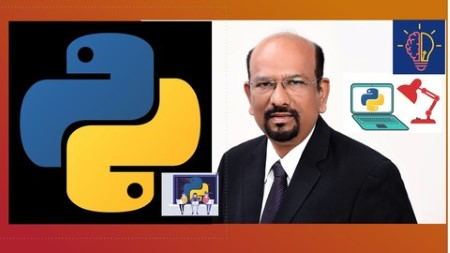Python Programming 2021 Full Coverage: A Practical Approach | UdemyPython Programming 2021 Full Coverage: A Practical Approach | Udemy
English | Size: 14.13 GB
Genre: eLearning

What you’ll learn
Python Programming
Develop a program for a given problem
Python Syntax and important Libraries
Python Image Library(PIL)
Data Visualization with Matplotlib
Play Music using Python library
Pandas Library
Math Library functions
Python NumPy library
Solutions for Python problems from University exam questions
Discussion on Job Interview questions on Python and related topics

In this course, we teach Python Language using a practical approach. For every concept and syntax, elaborate details are given with a practical example. For every topic, we introduce the required. level of theory, explain the syntax, and illustrate using several example programs. The Tutorial Videos give coaching on Job Interview questions and solutions for problems from university examination papers. The following topics first with a sufficient number of practical examples:

1. Introduction and basics of to Python

2. Assignment Statements

3. Input Statement and Output Statements

4. Math Library functions

5. Datetime Library – date, time, datetime, timedelta

6. Control structures such as IF statement

7. Loop Structures such as For Loop and While Loop

8. List, Tuple, and Set

9. String Manipulation

10. User-Defined Functions

11. Data Files – File Manipulation

12. Drawing with Python Image Library (PIL)

13. Data Visualization using Matplotlib

14. Database Management using Python Pandas Data Frames

15. Playing Music with Python programs

We introduce some important Python Libraries such as Math, Pandas, Matplotlib, NumPy, and PIL.

Examples are chosen for each of the concepts so that the student understands the concept easily. In Math Library, we introduce all the important Functions such as Math constants, number functions, trigonometric functions, logarithmic functions, distance, permutation combination, and LCM / GCD. In introducing Pandas Dataframe, we have used a simple database and explained all the data frame methods and commands. By introducing PIL, we have illustrated the library capabilities such as load and display a picture, Image processing operations such as Sharp, Blur, and embodying. We have written programs for drawing rectangles and other shapes on a picture. We have used PIL.ImageFont library to write text on the image.

In Matplotlib, we have introduced Python programs for drawing line graphs, bar charts, scatter diagrams, horizontal bars, and pie chart.

We have added Tutorial videos for discussing the solutions for problems asked in university examinations globally.

Who this course is for:
Programmers
Students of Computer Science and Engineering, Mathematics, Physics, Statistics, Other Engineering
IT Professionals, College and High School Students
Software Developers
Bright Children who aspire to become Software professionalsIf any links die or problem unrar, send request to
goo.gl/t4uR9GAbout WoW Team

I'm WoW Team , I love to share all the video tutorials. If you have a video tutorial, please send me, I'll post on my website. Because knowledge is not limited to, irrespective of qualifications, people join hands to help me.

This site uses Akismet to reduce spam. Learn how your comment data is processed.